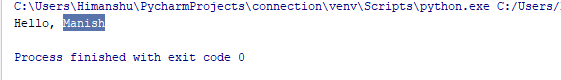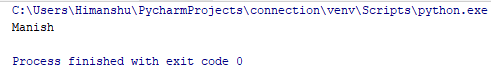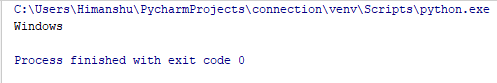×

### Html & Html5

Introduction to Html

Component of Html

Html Form

Html5 basics

Html5 Grid

Html5 Graphics

Html5 Media

Html5 APIs

### Python and Pandas

Introduction to Python

Component of Python

### Python Module

Module is just a code library in which we set our functions which we want to include in our applications.
Let's understand this by creating a module :-

Creating a module

We can create a module just by saving the code we want with the file extension .py

def greeting(name):

print("Hello, " + name)

We must save this file with .py extension. As we are here saving this file with msbb.py extension.

Use a module

Now we will understand how to use this module by just using the import statement . We can use this above module in any of our other python project file.
So let’s make it clear through the following example :-

import msbb

msbb.greeting("Manish")

### Output of this ProgramHere we have used our msbb module in our new project file.

Variable in module

We can create those module which can contain all types of variables like (array, dictionary,tuple etc.):
Let's understand this by the following program :-

person = { "name": "Manish","age": 21,"country": "India" }

As here also we save this file with msbb.py extension.

Now as above we have created our module now we will use it in our different project file.
Let’s understand how :-

import msbb

a = msbb.person["name"]

print(a)

And this file we have saved with nikki.py extension . Now let’s check out the result

### Output of this ProgramSo here we have used variables in module.

Built-in module

Here in Python several predefined in-built modules are there which we can use too and these predefined built-in modules have predefined functions too.
Let's understand this by the following program :-

import platform

x = platform.system()

print(x)

### Output of this ProgramSo here we are using the platform built-in module which just tell us the platform which we are using, and here it tells us that the platform is windows.

python training insitute| Best IT Training classes in Gurgaon | python training